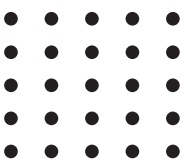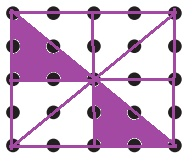Home | | Maths 4th Std | Symbolic Representation of Simple Fraction

# Symbolic Representation of Simple Fraction

Relating parts to whole eg: filling up water in a measured bottle partially, fixing up, puzzels circularly, vertically, horizontally in places and completes the whole.

UNIT – 6

FRACTIONSSymbolic Representation of Simple Fraction:

• Relating parts to whole eg: filling up water in a measured bottle partially, fixing up, puzzels circularly, vertically, horizontally in places and completes the whole.

Introduction

Part and parts:Rani andGowri wants equal share from 4 dosas. Could you please suggest an idea?

How many dosas are there?How many people are there?

Two

So, how many shares have to be done for each?So, Rani will get 2 out of 4 dosas and Gowri will get 2 out of 4 dosas.

Activity 1

Divide the following figure into different segments. (either vertically or horizontally)There are 8 equal parts.

Shade as many parts as you wish. It can be written as 2 out of8 parts are shaded.

Activity 2Draw lines using the dots above. It could be vertically/horizontally/ diagonally.

There are equal parts. Shade as many parts as you wish. It can be written as 2 out of 8 parts are shaded.

Activity 3

(The four equal parts of a circle)

A Circle which is divided into four equal parts are given to Arun, Tharun, sankar and Gopi, to colour it.

They could complete the task of colouring in two minutes, which is given below.In the above pictures one fourth (quarter) portion was coloured by tharun.

1/2 portion was coloured by Arun

Whole portion was coloured by Sankar

Three fourth of the portion was coloured by Gopi

Identify half, one fourth, and three fourths of a whole.The above pictures were divided into two equal halves.The above shapes are divided into two equal parts. In the two equal parts, one part was shaded. The shaded portion was one half and the unshaded part was another half.Half of 8 is 4.

The above collection of whole was divided into two equal parts.

Shade the following figure as per the fractions mentioned above them. One is done for you.Tags : Fractions | Term 3 Chapter 6 | 4th Maths , 4th Maths : Term 3 Unit 6 : Fractions
Study Material, Lecturing Notes, Assignment, Reference, Wiki description explanation, brief detail
4th Maths : Term 3 Unit 6 : Fractions : Symbolic Representation of Simple Fraction | Fractions | Term 3 Chapter 6 | 4th Maths